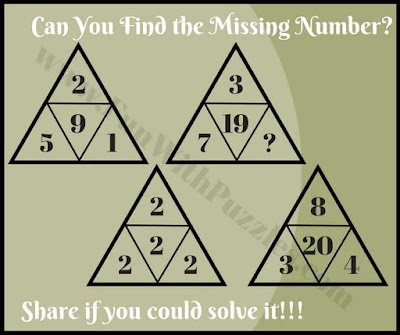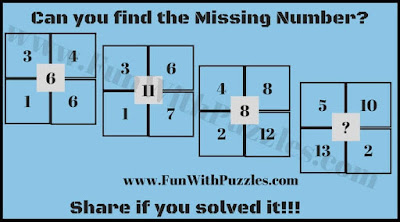Mathematics Brain Teasers are very much fun to solve. Maths Brain Teasers come with many different cool variations like Numbers around the shapes Maths Puzzles in which one has to find the missing number which will replace the question mark. There are also very cool Maths Equations Brain Teasers in which one has to solve some Maths Equations in which variables are represented by different geometrical shapes.
In these Cool Maths Brain Teasers, there are groups of few numbers which has a logical relationship with each other. Your challenge is to find this Logical relationship and then solve for the missing number which will replace the question mark.
Answers along with explanations to these Cool Maths Brain Teasers are given at the end. Also, links to the posts containing similar Maths Brain Teasers are given after each of the Picture Puzzles Images. Do click on these links to solve similar Maths Brain Teasers.1. Maths Logical Riddle2. Logical Maths Quick Brain Teaser3. Triangles Maths Brain Teaser4. Tricky Math Riddle

Last Maths Brain Teaser is Simple Maths equations in which variables are replaced with geometrical shapes. Your task is to solve these equations and find the values of these geometrical shapes.5. Geometric Shapes Math Equations Riddle

Answers and Explanations of these Cool Maths Brain Teasers are given below

A1) 2,
5x2-1 = 9, 2x2-2=2, 3x8-4=20,Similarly 7x3-2=19.

A2) 14
Let's say A  B = X
Here Logical Reasoning is A*B/2 = X
So 7*4/2 = 14

A3) 126.
Required number=  multiplication of the three numbers on the three sides of the triangle.

A4)  24.
Required number =multiplication of the numbers on the upper side -  multiplication of the numbers on the lower side.
Required number =10x5 - 13x2=24

A5)
Triangle = 4 , Circle = 5,   Square= 3

triangle = 4 , circle = 5,   square= 3.,
explanation is same way as in question 4.